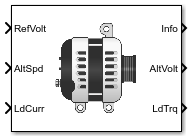# Reduced Lundell Alternator

Reduced Lundell (claw-pole) alternator with an external voltage regulator

• Library:
• Powertrain Blockset / Energy Storage and Auxiliary Drive / Alternator

•## Description

The Reduced Lundell Alternator block implements a reduced Lundell (claw-pole) alternator with an external voltage regulator. The back-electromotive force (EMF) voltage is proportional to the input velocity and field current. The motor operates as a source torque to the internal combustion engine.

Use the Reduced Lundell Alternator block:

• To model an automotive electrical system

• In an engine model with a front-end accessory drive (FEAD)

The calculated motor shaft torque is in the opposite direction of the engine speed. You can:

• Tune the external voltage regulator to a desired bandwidth. The stator current and two diode drops reduce the stator voltage.

• Filter the load current to desired bandwidth. The load current has a lower saturation of 0 A.

The Reduced Lundell Alternator block implements equations for the electrical, control, and mechanical systems that use these variables.

### Electrical

To calculate voltages, the block uses these equations.

CalculationEquations
Alternator output voltage${v}_{s}={K}_{v}{i}_{f}\omega -{R}_{s}{i}_{s}-2{V}_{d}$
Field winding voltage

### Control

The controller assumes no resistance or voltage drop.

CalculationEquations

Field winding voltage transform

${V}_{f}\left(s\right)={R}_{f}{I}_{f}\left(s\right)+s{L}_{f}{I}_{f}\left(s\right)$

Field winding current transform

${I}_{f}\left(s\right)=\frac{{V}_{f}\left(s\right)}{\left({R}_{f}+s{L}_{f}\right)}$

Open loop electrical transfer function

Open loop voltage regulator transfer function

Closed loop transfer function

Closed loop controller design

### Mechanical

To calculate torques, the block uses these equations.

CalculationEquations
Electrical torque

Frictional torque

Windage torque

${\tau }_{windage}={K}_{w}{\omega }^{2}$

Torque at start

${\tau }_{start}={K}_{c}$ when $\omega =0$

Motor shaft torque

${\tau }_{mech}={\tau }_{elec}+{\tau }_{friction}+{\tau }_{windage}+{\tau }_{start}$

### Power Accounting

For the power accounting, the block implements these equations.

Bus Signal DescriptionVariableEquations

`PwrInfo`

`PwrTrnsfrd` — Power transferred between blocks

• Positive signals indicate flow into block

• Negative signals indicate flow out of block

`PwrMtr`

Mechanical power

Pmot

`PwrBus`

Electrical power

Pbus

`PwrNotTrnsfrd` — Power crossing the block boundary, but not transferred

• Positive signals indicate an input

• Negative signals indicate a loss

`PwrLoss`

Motor power loss

Ploss

`PwrStored` — Stored energy rate of change

• Positive signals indicate an increase

• Negative signals indicate a decrease

`PwrInd`

Electrical winding loss

Pind

The equations use these variables.

 vref Alternator output voltage command vf Field winding voltage if Field winding current is Stator winding current Vd Diode voltage drop Rf Field winding resistance Rs Stator winding resistance Lf Field winding inductance Kv Voltage constant Fv Voltage regulator bandwidth Fc Input current filter bandwidth Vfmax Field control voltage upper saturation limit Vfmin Field control voltage lower saturation limit Kc Coulomb friction coefficient Kb Viscous friction coefficient Kw Windage coefficient ω Motor shaft angular speed iload Alternator load current vs Alternator output voltage τmech, Tmech Motor shaft torque

## Ports

### Inputs

expand all

Alternator output voltage command, in V.

Motor shaft input angular speed, in rad/s.

Do not connect the port to the alternator rated current, which is a constant value. The block uses the alternator load current as the stator winding current, is, to determine the alternator voltage and motor torque. If you connect the port to the rated alternator current, the block does not model the dynamic effect of load current changes on the voltage and motor torque.

### Output

expand all

Bus signal containing these block calculations.

SignalDescriptionUnits

`FldVolt`

Field winding voltage

A

`FldFlux`

Field flux

Wb

`PwrInfo`

`PwrTrnsfrd`

`PwrMtr`

Mechanical power

W
`PwrBus`

Electrical power

W

`PwrNotTrnsfrd`

`PwrLoss`

Motor power loss

W

`PwrStored`

`PwrInd`

Electrical winding loss

W

Alternator output voltage, in V.

Motor shaft torque, in N·m.

## Parameters

expand all

Machine Configuration

Field winding resistance, in ohm.

Field winding inductance, in H.

Stator winding resistance, in ohm.

Diode voltage drop, in V.

Voltage Regulator

The regulator bandwidth, in Hz.

The current filter bandwidth, in Hz.

The maximum field voltage, in V.

The minimum field voltage, in V.

Mechanical Losses

Coulomb friction, in N·m.